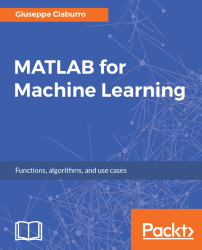•#### MATLAB for Machine Learning#### Overview of this book

MATLAB is the language of choice for many researchers and mathematics experts for machine learning. This book will help you build a foundation in machine learning using MATLAB for beginners. You’ll start by getting your system ready with t he MATLAB environment for machine learning and you’ll see how to easily interact with the Matlab workspace. We’ll then move on to data cleansing, mining and analyzing various data types in machine learning and you’ll see how to display data values on a plot. Next, you’ll get to know about the different types of regression techniques and how to apply them to your data using the MATLAB functions. You’ll understand the basic concepts of neural networks and perform data fitting, pattern recognition, and clustering analysis. Finally, you’ll explore feature selection and extraction techniques for dimensionality reduction for performance improvement. At the end of the book, you will learn to put it all together into real-world cases covering major machine learning algorithms and be comfortable in performing machine learning with MATLAB.
Title PageCreditsForewordwww.PacktPub.comCustomer FeedbackPrefaceFree Chapter
Getting Started with MATLAB Machine LearningImporting and Organizing Data in MATLABFrom Data to Knowledge DiscoveryFinding Relationships between Variables - Regression TechniquesPattern Recognition through Classification AlgorithmsIdentifying Groups of Data Using Clustering MethodsSimulation of Human Thinking - Artificial Neural NetworksImproving the Performance of the Machine Learning Model - Dimensionality ReductionMachine Learning in Practice## Data preparation

Once data collection has been completed and imported into MATLAB, it is finally time to start the analysis process. This is what a novice might think; conversely, we must first proceed to the preparation of data (data wrangling). This is a laborious process that can take a long time, in some cases about 80 percent of the entire data analysis process. However, it is a fundamental prerequisite for the rest of the data analysis workflow, so it is essential to acquire the best practices in such techniques.

Before submitting our data to any machine learning algorithm, we must be able to evaluate the quality and accuracy of our observations. If we cannot access the data stored in MATLAB correctly or if we do not know how to switch from raw data to something that can be analyzed, we cannot go ahead.

### A first look at data

Before passing our data to machine learning algorithms, we need to give a first look at what we've imported into MATLAB to see if there are any issues. Often, raw...